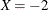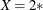### UNITS Statement

`UNITS` independent1 = list1 <…independentk = listk> </ option> ;

The UNITS statement enables you to specify units of change for the continuous explanatory variables so that customized odds ratios can be estimated. An estimate of the corresponding odds ratio is produced for each unit of change specified for an explanatory variable. The UNITS statement is ignored for CLASS variables. If the CLODDS option is specified in the MODEL statement, the corresponding confidence limits for the odds ratios are also displayed.

The term independent is the name of an explanatory variable, and list represents a list of units of change, separated by spaces, that are of interest for that variable. Each unit of change in a list has one of the following forms:

• number

• SD or –SD

• number * SD

where number is any nonzero number and SD is the sample standard deviation of the corresponding independent variable. For example,requests an odds ratio that represents the change in the odds when the variable X is decreased by two units.SD requests an estimate of the change in the odds when X is increased by two sample standard deviations.

You can specify the following option in the UNITS statement after a slash (/):

DEFAULT=list

gives a list of units of change for all explanatory variables that are not specified in the UNITS statement. Each unit of change can be in any of the forms described previously. If the DEFAULT= option is not specified, PROC SURVEYLOGISTIC does not produce customized odds ratio estimates for any explanatory variable that is not listed in the UNITS statement.